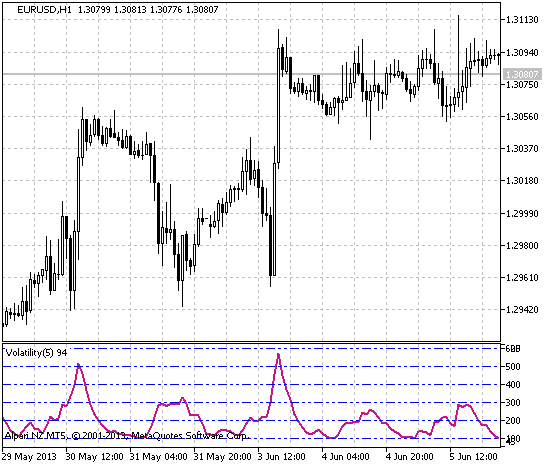Interesting script?
So post a link to it -
let others appraise it
You liked the script? Try it in the MetaTrader 5 terminal# Volatility2 - indicator for MetaTrader 5

|
Views:
6612
Rating:
(25)
Published:
2013.07.30 21:41
Updated:
2016.11.22 07:32

Real author:

Eva Ruft

A simple indicator that calculates only the volatility of a financial asset. Volatility is calculated in points based on the maximum and minimum prices.

The volatility is calculated using a simple MA as the sum of the maximum prices for the averaging period minus the sum of minimal prices for the same period. The resulting value is calculated in points.

Indicator input parameters:

```//+-----------------------------------+
//|  INDICATOR INPUT PARAMETERS       |
//+-----------------------------------+
input  ENUM_MA_METHOD   MAType=MODE_SMA;     // Method of averaging
input uint              VolatilityPeriod=5;  // Indicator period
input int               Shift=0;             // Horizontal shift of the indicator in bars

input uint              LevelsTotal=20;      // Number of levels
input uint              StartLevel=100;      // Starting level
input uint              LevelsStep=100;      // Distance between levels
input color             LevelsColor=clrBlue; // Color of the levels```

This indicator was first implemented in MQL4 and published in Code Base at mql4.com on 08.04.2008.Figure 1. The Volatility2 indicator

Translated from Russian by MetaQuotes Ltd.
Original code: https://www.mql5.com/ru/code/1763ATRStops_v1

A trend indicator implemented in the form of NRTRChange To Range Ratio

The Change To Range Ratio indicator is used for closing short-term positions and/or for filtering entry points.ATRStops_v1_HTF

A trend indicator implemented in the form of NRTR. Timeframe is specified in the input parameters of the indicator.PinBar

An indicator of pin bars, to be used as a built-in indicator in Expert Advisors. Shows levels specified by the pattern for the open price and stop-loss.